Controllers are used to interface FitBenchmarking with the various fitting packages. Controllers are responsible for converting the problem into a format that the fitting software can use, and converting the result back to a standardised format (numpy arrays). As well as this, the controller must be written so that the fitting is separated from the preparation wherever possible in order to give accurate timings for the fitting. Supported controllers are found in fitbenchmarking/controllers/.

In order to add a new controller, you will need to:

1. Give the software a name <software_name>. This will be used by users when selecting this software.
2. Create fitbenchmarking/controllers/<software_name>_controller.py which contains a new subclass of Controller. This should implement four functions:
• Controller.__init__()

Initialise anything that is needed specifically for the software, do any work that can be done without knowledge of the minimizer to use, or function to fit, and call super(<software_name>Controller, self).__init__(problem) (the base class’s __init__ implementation). In this function, you must initialize the a dictionary, self.algorithm_type, such that the keys are given by:

• all - all minimizers
• ls - least-squares fitting algorithms
• deriv_free - derivative free algorithms (these are algorithms that cannot use derivative information. For example, the Simplex method in Mantid does not require Jacobians, and so is derivative free. However, lm-scipy-no-jac in scipy_ls is designed to use derivatives, but calculates an approximation internally if one is not supplied.)
• general - minimizers which solve a generic min f(x).

The values of the dictionary are given as a list of minimizers for that specific controller that fit into each of the above categories. See for example the GSL controller.

Parameters: problem (FittingProblem) – The parsed problem
• Controller.setup()

Setup the specifics of the fitting.

Anything needed for “fit” that can only be done after knowing the minimizer to use and the function to fit should be done here. Any variables needed should be saved to self (as class attributes).

• Controller.fit()

Run the fitting.

This will be timed so should include only what is needed to fit the data.

• Controller.cleanup()

Retrieve the result as a numpy array and store results.

Convert the fitted parameters into a numpy array, saved to self.final_params, and store the error flag as self.flag.

The flag corresponds to the following messages:

flag()
0: Successfully converged
1: Software reported maximum number of iterations exceeded
2: Software run but didn’t converge to solution
3: Software raised an exception
1. Add the new software to the default options, following the instructions in Adding new Options.

Your new software is now fully hooked in with FitBenchmarking, and you can compare it with the current software. You are encouraged to contribute this to the repository so that other can use this package. To do this need to follow our Coding Standards and our Git Workflow, and you’ll also need to

1. Document the available minimizers (see Fitting Options, Minimizer Options). Note: make sure that you use <software_name> in these places so that the software links in the HTML tables link correctly to the documentation. Add the software to examples/all_software.ini.
2. Create tests for the software in fitbenchmarking/controllers/tests/test_controllers.py. If the package is pip installable then add the tests to the DefaultControllerTests class and if not add to the ExternalControllerTests class. Unless the new controller is more complicated than the currently available controllers, this can be done by following the example of the others.
3. If pip installable add to install_requires in setup.py otherwise document the installation procedure in Installing External Software. Update the FullInstall Docker Container – the main developers will help you with this step.

Note

For ease of maintenance, please add new controllers to a list of software in alphabetical order.

## The FittingProblem and Jacobian classes¶

When adding new minimizers, you will find it helpful to make use of the following members of the FittingProblem and subclasses of Jacobian classes:

class fitbenchmarking.jacobian.base_jacobian.Jacobian(problem)

Base class for Jacobian.

eval(params, func=None, **kwargs)

Evaluates Jacobian

Parameters: params (list) – The parameter values to find the Jacobian at func (Callable, optional) – Function to find the Jacobian for, defaults to problem.eval_r Approximation of the Jacobian numpy array
class fitbenchmarking.parsing.fitting_problem.FittingProblem(options)

Definition of a fitting problem, which will be populated by a parser from a problem definition file.

This defines a fitting problem where, given a set of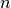data points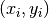, associated errors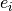, and a model function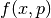, we find the optimal parameters in the least-squares sense by solving:where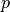is a vector of length, and we start from a given intial guess for the optimal parameters.

data_e = None

numpy array The errors

data_x = None

numpy array The x-data

data_y = None

numpy array The y-data

eval_f(params, x=None)

Function evaluation method

Parameters: params (list) – parameter value(s) x (numpy array) – x data values or None, if None this uses self.data_x y data values evaluated from the function of the problem numpy array
eval_r(params, x=None, y=None, e=None)

Calculate residuals and weight them if using errors

Parameters: params (list) – The parameters to calculate residuals for x (numpy array, optional) – x data points, defaults to self.data_x y (numpy array, optional) – y data points, defaults to self.data_y e (numpy array, optional) – error at each data point, defaults to self.data_e The residuals for the datapoints at the given parameters numpy array
eval_r_norm(params, x=None, y=None, e=None)

Evaluate the square of the L2 norm of the residuals

Parameters: params (list) – The parameters to calculate residuals for x (numpy array, optional) – x data points, defaults to self.data_x y (numpy array, optional) – y data points, defaults to self.data_y e (numpy array, optional) – error at each data point, defaults to self.data_e The sum of squares of residuals for the datapoints at the given parameters numpy array
starting_values = None

list of dict Starting values of the fitting parameters

e.g. [{p1_name: p1_val1, p2_name: p2_val1, ...}, {p1_name: p1_val2, ...}, ...]